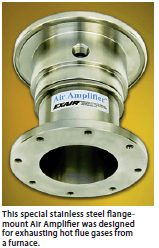My colleague, Lee Evans, wrote a blog about calculating the size of receiver tanks within a compressor air system.  (You can read it here: Receiver Tank Principle and Calculations).  But, what if you want to use them in remote areas or in emergency cases?  During these situations, the air compressor is not putting any additional compressed air into the tank.  But, we still have potential energy stored inside the tanks similar to a capacitor that has stored voltage in an electrical system.  In this blog, I will show how you can calculate the size of receiver tanks for applications that are remote or for emergency systems.

From Lee Evans’ blog, Equation 1 can be adjusted to remove the input capacity from an air compressor.  This value is Cap below.  During air compressor shutdowns or after being filled and removed, this value becomes zero.

Receiver tank capacity formula (Equation 1)

V = T * (C – Cap) * (Pa) / (P1-P2)

V – Volume of receiver tank (cubic feet)

T – Time interval (minutes)

C – Air requirement of demand (cubic feet per minute)

Cap – Compressor capacity (cubic feet per minute)

Pa – Absolute atmospheric pressure (PSIA)

P1 – Tank pressure (PSIG)

P2 = minimum tank pressure (PSIG)

Making Cap = 0, the new equation for this type of receiver tank now becomes Equation 2.

Receiver tank capacity formula (Equation 2)

V = T * C * (Pa) / (P1-P2)

With Equation 2, we can calculate the required volume of a receiver tank after it has been pre-charged.  For example, EXAIR created a special Air Amplifier to remove toxic fumes from an oven.  The Air Amplifier was positioned in the exhaust stack and would only operate during power failures.  In this situation, product was being baked in an oven.  The material had toxic chemicals that had to cross-link to harden.  If the power would go out, then the product in the oven would be discarded, but the toxic fumes had to be removed.  What also doesn’t work during power outages is the air compressor.  So, they needed to have a receiver tank with enough volume to store compressed air.  From the volume of the oven, we calculated that they need the special Air Amplifier to operate for 6 minutes.  The compressed air system was operating at 110 PSIG, and the Air Amplifier required an average air flow of 10 cubic feet per minute from the range of 110 PSIG to 0 PSIG.  We are able to calculate the required receiver volume to ensure that the toxic fumes are evacuated from the oven in Equation 2.Receiver tank capacity formula (Equation 2)

V = T * C * Pa / (P1 – P2)

V = 6 minutes * 10 cubic feet per minute * 14.7 PSIA / (110 PSIG – 0 PSIG)

V = 8 cubic feet.

Receiver tanks are more commonly sized in gallons.  In converting 8 cubic feet to gallons, we get a 60-Gallon Receiver Tank.  EXAIR recommended the model 9500-60 to be used near the oven to operate the special Air Amplifier during power outage.

Another way to look at Equation 2 is to create a timing equation.  If the volume of the tank is known, we can calculate how long a system will last.  In this example for scuba diving, we can use this information to configure the amount of time that a tank will last.  The diver has a 0.39 cubic feet tank at a pressure of 3,000 PSIG.  I will use a standard Surface Consumption Rate, SCR, at 0.8 cubic feet per minute.  If we stop the test when the tank reaches a pressure of 1,000 PSIG, we can calculate the time by using Equation 3.

Receiver tank timing formula (Equation 3):

T = V * (P1 – P2) / (C * Pa)

T – Time interval (minutes)

V – Volume of receiver tank (cubic feet)

C – Air demand (cubic feet per minute)

Pa – Absolute atmospheric pressure (PSIA)

P1 – Initial tank pressure (PSIG)

P2 – Ending tank pressure (PSIG)

By placing the values in the Equation 3, we can calculate the time to go from 3,000 PSIG to 1,000 PSIG by breathing normal at the surface.

T = 0.39 cubic feet * (3,000 PSIG – 1,000 PSIG) / (0.8 cubic feet per minute * 14.7 PSIA)

T = 66 minutes.

What happens if the diver goes into deeper water?  The atmospheric pressure, Pa, changes.  If the diver goes to 100 feet below the surface, this is roughly 3 atmospheres or (3 * 14.7) = 44.1 PSIA.  If we use the same conditions above except at 100 feet below, the time will change by a third, or in looking at Equation 3:

T = 0.39 cubic feet * (3,000 PSIG – 1,000 PSIG) / (0.8 cubic feet per minute * 44.1 PSIA)

T = 22 minutes.

If you have any questions about using a receiver tank in your application, you can contact an EXAIR Application Engineer.  We will be happy to solve for the proper volume or time needed for your application.

John Ball
Application Engineer
Email: johnball@exair.com